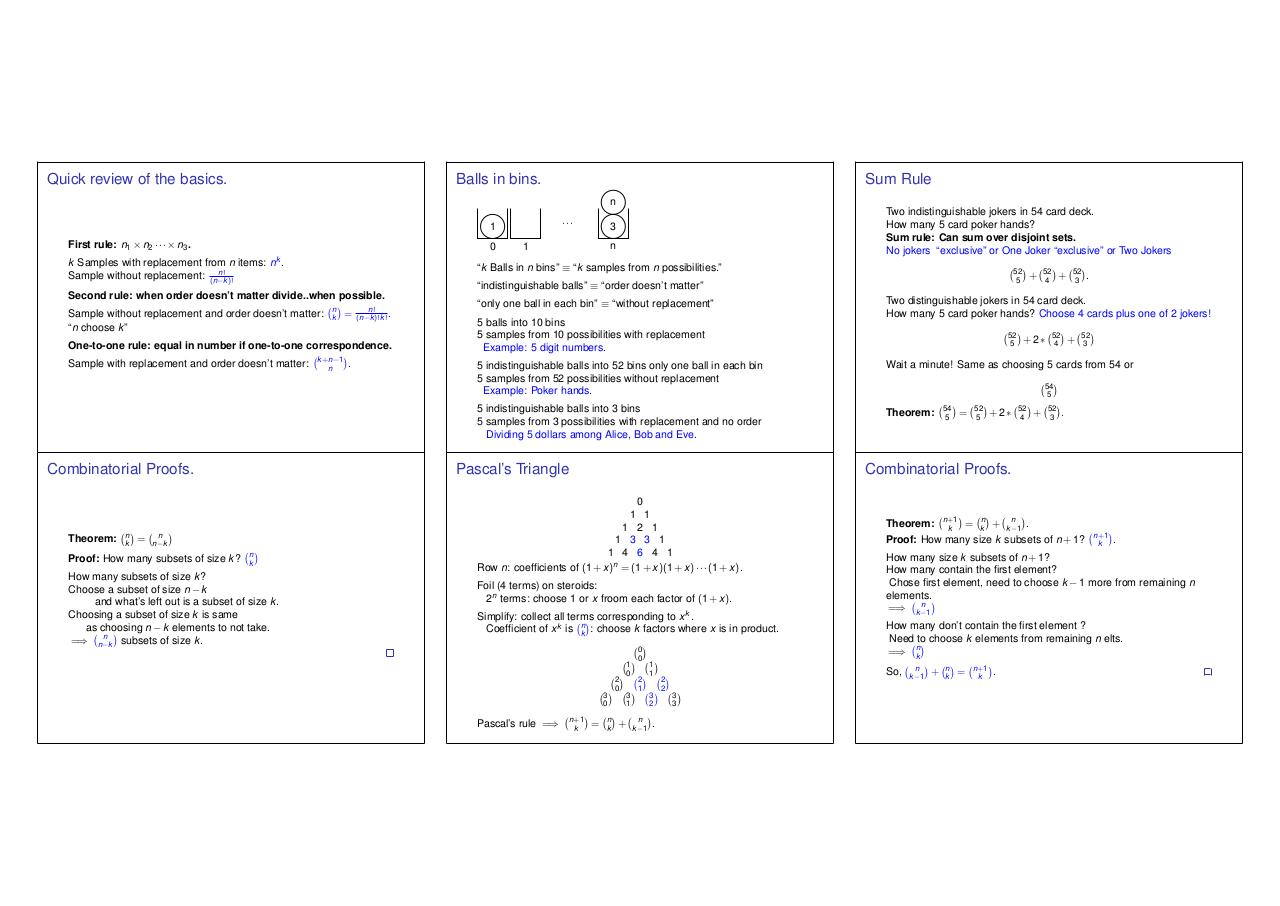# lec 15.6up.pdfPage 1 2 3 4 5 6 7

#### Text preview

Quick review of the basics.

Balls in bins.

Sum Rule
n
···

1
First rule: n1 ⇥ n2 · · · ⇥ n3 .

0

k Samples with replacement from n items: nk .
Sample without replacement: (n n!k )!
Sample without replacement and order doesn’t matter:
“n choose k”

n
k

=

n!
(n k )!k ! .

One-to-one rule: equal in number if one-to-one correspondence.

Combinatorial Proofs.

Theorem:

n
k

=

k +n 1
n

.

“indistinguishable balls” ⌘ “order doesn’t matter”

“only one ball in each bin” ⌘ “without replacement”
5 balls into 10 bins
5 samples from 10 possibilities with replacement
Example: 5 digit numbers.

n k
n
k

How many subsets of size k?
Choose a subset of size n k
and what’s left out is a subset of size k .
Choosing a subset of size k is same
as choosing n k elements to not take.
=) n n k subsets of size k .

52
5

+

52
4

52
3

+

.

Two distinguishable jokers in 54 card deck.
How many 5 card poker hands? Choose 4 cards plus one of 2 jokers!
52
5

+2⇤

52
4

+

52
3

5 indistinguishable balls into 52 bins only one ball in each bin
5 samples from 52 possibilities without replacement
Example: Poker hands.

Wait a minute! Same as choosing 5 cards from 54 or

5 indistinguishable balls into 3 bins
5 samples from 3 possibilities with replacement and no order
Dividing 5 dollars among Alice, Bob and Eve.

Theorem:

Pascal’s Triangle

n

Proof: How many subsets of size k ?

n

1

“k Balls in n bins” ⌘ “k samples from n possibilities.”

Second rule: when order doesn’t matter divide..when possible.

Sample with replacement and order doesn’t matter:

Two indistinguishable jokers in 54 card deck.
How many 5 card poker hands?
Sum rule: Can sum over disjoint sets.
No jokers “exclusive” or One Joker “exclusive” or Two Jokers

3

Foil (4 terms) on steroids:
2n terms: choose 1 or x froom each factor of (1 + x).

Simplify: collect all terms corresponding to x k .
Coefficient of x k is kn : choose k factors where x is in product.

3
0
n +1
k

54
5

=

52
5

+2⇤

52
4

+

52
3

.

Combinatorial Proofs.

0
1 1
1 2 1
1 3 3 1
1 4 6 4 1
Row n: coefficients of (1 + x)n = (1 + x)(1 + x) · · · (1 + x).

Pascal’s rule =)

54
5

=

n
k

2
0

+

1
0
3
1

0
0
2
1

n
k 1

1
1
3
2

.

2
2

1
Theorem: n+
= kn + k n 1 .
k
Proof: How many size k subsets of n + 1?

How many size k subsets of n + 1?
How many contain the first element?
Chose first element, need to choose k
elements.
=) k n 1

.

1 more from remaining n

How many don’t contain the first element ?
Need to choose k elements from remaining n elts.
=) kn
So,

3
3

n +1
k

n
k 1

+

n
k

=

n +1
k

.